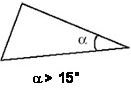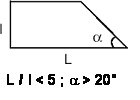# A valid mesh: some rules to follow

## Introduction

Quality of results depends on mesh element quality. Some recommendations on how to obtain a good quality mesh in Flux are described in the following blocks.

## General rules

We can establish some general rules:

• The finite elements should be “well proportioned” . The ideal elements for a surface mesh are equilateral triangles and squares . The ideal elements for a volume mesh are regular tetrahedrons and cubes . However, thanks to the second-order transformation used, the elements can be deformed within certain limits. The above table describes the geometry quality criteria for face elements (triangle, quadrangle).
 TriangleQuadrangle• The mesh should not be unnecessarily fine. A fine mesh requires a longer computation time. One may need to find a compromise between an accurate geometrical representation of the study domain and a reasonable computation time .
• Mesh elements located close to the study domain limits (i.e. close to the Infinite box) can be more relaxed.
• To mesh a complex shaped domain is an iterative process. One should try to utilize the available mesh tools in order to obtain a satisfying result.

## Mesh and the physics of the problem

It is necessary to adapt the mesh to the physics of the problem, as much as possible. The mesh refinement depends on the geometrical constraints , e.g., the mesh of a very thin region, but also on the physical constraints of the problem, such as a high variation in the permeability within an element, skin depth, etc.

As a general rule, a strong variation of the state variable requires the use of smaller elements.

When one has some idea about the final result, he can decide on a relaxed mesh in certain regions and a fine mesh in others. Analysis of the computation results may lead one to restart the computation with a new and better-adapted mesh.

Thus, one should always consider the mesh while the geometry is being constructed .

## Examples of criteria to validate a mesh

Different physics criteria may be used to validate a mesh. One can verify the following points:

• Once solved, if the field lines form cracks in the same region, the neighboring elements are too large.
• On a rotating machine, if the reaction force is different from the action force, the mesh on the air-gap region should be refined for more accurate precision.
• With circuit equations, if the current through a coil computed by several methods differs significantly, the mesh on the coil region should be refined.
• The mesh of a solid conductor must contain at least two element in the skin depth (cf Mesh of solid conductor with skin effect ).